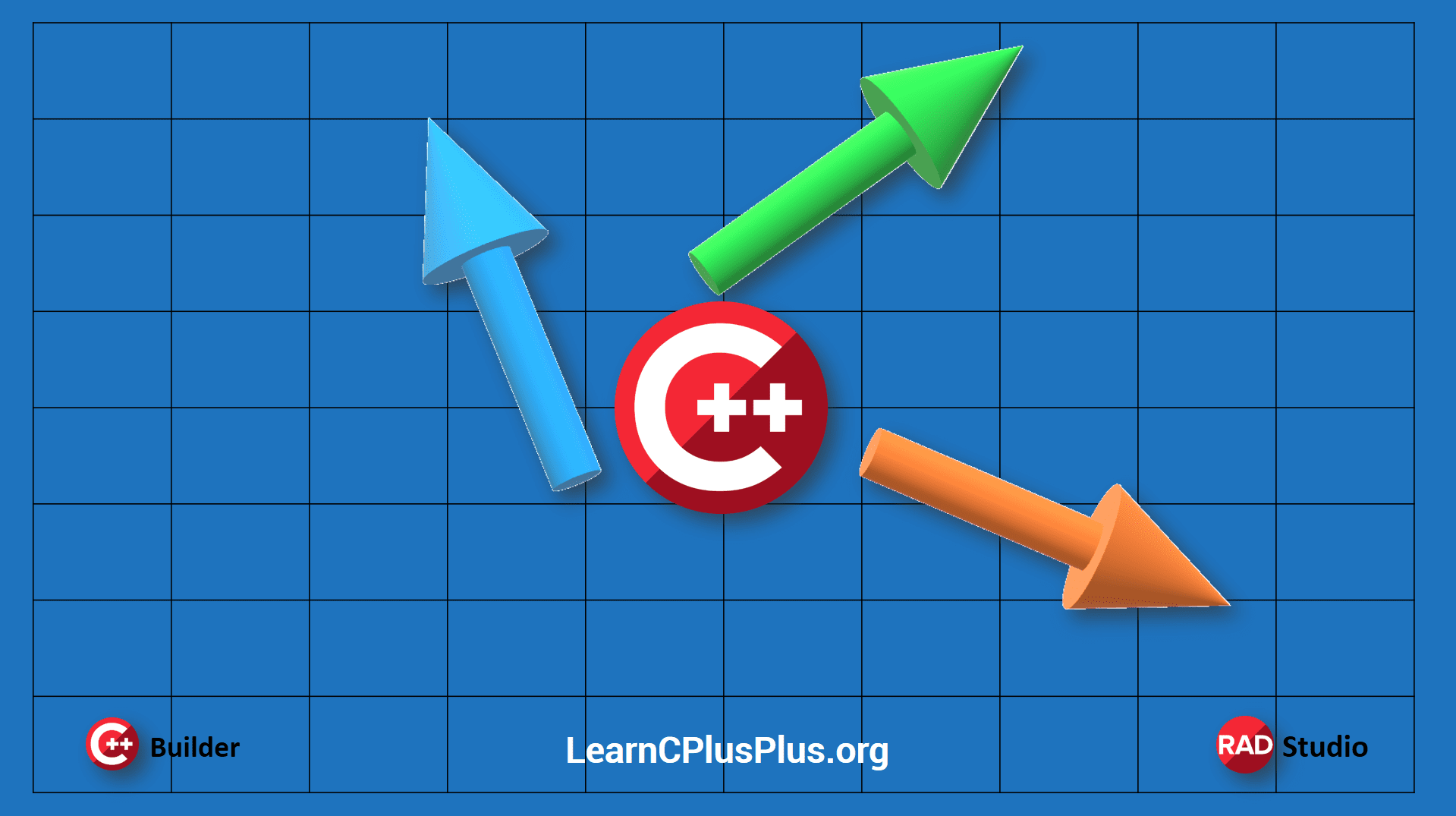# Why You Should Learn About Hilarious Hedgehogs In C++I know what you’re thinking: hedgehogs. Those cute, spiky little mammals who snuffle around in bushes and famously curl into a ball when life looks a little too scary. But, bear with me, those hedgehogs can serve a serious purpose in C++.

Do you want to draw vectors in arrows? Want to visualize with hedgehogs on a bitmap? In this post, we will explain how you can draw your engineering data in vector forms.

## Vector data is a multi-dimensional visualization problem for developers

In engineering mostly we have problems to solve in 1D, 2D, or 3D, and analysis results bring us a lot of data which are about a line, a plane or a 3D object. In some cases, We should see the direction of each data with their magnitude. Vector data can be a one-dimensional, two-dimensional or three-dimensional representation of direction and magnitude. In that case, we can use vector visualization.

This data generally from the study of fluid flow, or when examining derivatives, i.e. rate of change, of some quantity. Sometimes it is hard to find specific data visualization components for those kinds of advanced visualizations. Do you want to learn how to draw a vector, how we can visualize our two-dimensional data set in vectoral form with hedgehogs?

## There are different visualization techniques are available for vector data sets,

• Hedgehogs
• Oriented glyphs
• Warping
• Displacement plots
• Time animation
• Streamlines

Hedgehogs, is a term which describes a Vectoral Visualization technique which is often referred to as a hedgehog because of the hedgehog spike-like graphic results. In this method, we draw an oriented, scaled line for each vector data. Each line would have its origin at a data point and its orientation in the direction of the vector. The length of each line segment is scaled to represent the vector’s magnitude, but additional scaling (scale, arrow_scale) variables may be required to improve its visual appearance.

## For a two-dimensional data sets, there might be 3 different vector data sets,

1. Vectors with Start and End Points ( from x1,y1to to x2,y2)
2. Vectors with Magnitude and Angle ( v and alpha in order)
3. Vectors with Divergence (dx, dy in order)

Now let’s see how we can draw these vector arrows in C++ Builder VCL applications.

## 1. Vectors with Start and End Points

In this data set, vectors are exactly known well with their start and end points. For example in 2D plane these x and y data can be integer, float or we can use TPoint and TPointF for the points , for a 3D display it can be x1,y1,z1 and x2,y2,z2 or TPoint3D. This procedure below draws a line from x1,y1 to x2,y2 and arrow head at x2, y2.

## 2. Vectors with Magnitude and Angle

If you have magnitude (V) and angle (alpha) of vectors, we can draw this vector as below. Here we can point our x1, y1 on graphic or we can calculate x1 and y1 from the index of data (something like x1=index%width, Y1=index/depth).

## 3. Vectors with Divergence (u, v)

We can draw vector by using their dx, dy and dz divergence in X, Y and Z directions. Here below we used u for the dx and and v for the dy.

Now let’s see all of 3 examples on C++ Builder VCL application, To do this,

1. Create a new C++ Builder VCL Application for Windows. Save all project and unit files to a folder
2. Add an Image (Tımage) to display vectors and a Button (TButton) to draw in 3 types.

If we run by F9, each given data sets will have same results. Here you will see Y direction goes to down, if you want to display in mathematical X-Y plots you can multiply y direction divergences with -1. In this example above 3 different data sets are same vectors, here below you can see 3 different visualization of same vector data with 3 different functions.

## Here below is an old application example which runs on the latest RAD Studio 10.4.

This shows how those procedures can be used to display velocity of fluid in a rectangular channel flow.

Note that these draw_vectordata() drawing procedures are for learning and it is not optimized, these examples above can be optimized for faster results. We know that you can optimize this well. Now it is your turn 🙂 You can develop your own analyze applications with C++ Builder by using VCL or FMX frameworks and you can display your data. FMX has more options like using alpha colors, using opacity that may result better graphics. You can also add gradient colors these vectors to show their magnitude.